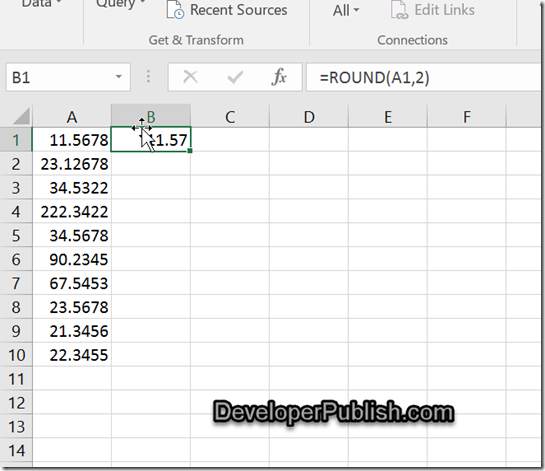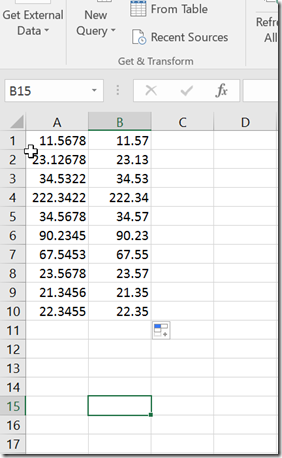# How to round the values in a range of cells in Microsoft Excel?

Assume that you have a range of cells that contains values with 4 decimal places and you wish to round their values to 2 decimal places in Microsoft Excel 2016.

# How to round the values in a range of cells in Microsoft Excel?

You can use the Excel’s function ROUND along with the auto-fill handle to achieve this.

The Syntax of the ROUND function looks like this

=ROUND(<number>, <digitsforroundoff>)

Assume that you have the cells A:A10 containing the values with 4 decimal places and you want to display the rounded value with 2 decimal places in the adjacent cells of column B.

1. Select the cell B1 and enter the formula =ROUND (A1, 2) and press the enter key.2. The value in the cell A1 would be rounded to the number with 2 decimal places and displayed in the cell B1.Now , Select the cell B1 and drag the fill handle over the range of cells (B1 to B10). You should immediately see the rounded off values in other selected cells as well.